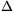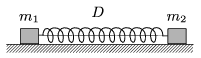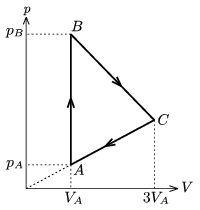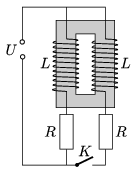Mathematical and Physical Journal
for High Schools
Issued by the MATFUND Foundation
 Already signed up? New to KöMaL?

# KöMaL Problems in Physics, February 2006

Show/hide problems of signs:## Problems with sign 'M'

Deadline expired on March 13, 2006.

M. 267. Place a cuboid-shaped, soft, dry sponge to a horizontal tabletop, such that its greatest face is on the table. Put a sheet of cardboard to the top of the sponge and load it gradually. How does the compression of the sponge depend on the load? Then gradually cease the loading. What can be observed? Repeat the experiment with sponge-balls.

(6 pont)

statistics## Problems with sign 'P'

Deadline expired on March 13, 2006.

P. 3864. It takes a certain time for a cylinder to slide down without slipping on an inclined plane with friction. Using energy methods decide whether the cylinder would slide down during shorter or longer time if there was no friction.

(3 pont)

statistics

P. 3865. The speed of an object undergoing uniform circular motion is 4 m/s. The magnitude of the change in the velocity during 0.5 is also 4 m/s. Find the angular velocity, and the centripetal acceleration of the object. What is the radius of the circle?

(4 pont)

statistics

P. 3866. Two small bodies of masses m1, and m2 are attached to the two ends of an ideal spring of spring constant D. The spring is stretched byl, and then the two bodies are released at the same time. Find the greatest speeds of the two masses, the amplitudes of their oscillations, and the frequencies. Friction is negligible. (Data: m1=1 kg, m2=2 kg, D=100 N/m,l=9 cm.)(4 pont)

statistics

P. 3867. An unstretchable string of uniform density and constant diameter is suspended at one of its ends and is hanging. At a length of L it breaks under its own weight. Is it conceivable that there is such shape of string which does not get broken under its own weight however long it is?

(5 pont)

statistics

P. 3868. Two similar retorts are connected with a glass tube, and there is a mercury drop in the middle of the glass tube. In one of the retorts there is helium gas and in the other one there is nitrogen. The temperature and the pressure of the gases are both equal. The whole system is placed into hot water and initially the mercury drop moves towards the left and then it goes back to the middle position. Which retort contains which gas?

(4 pont)

statistics

P. 3869. A sample of ideal gas is taken through the cyclic process shown in the figure. The temperature of the gas at state A is TA=200 K. At states B and C the temperature of the gas is the same.a) What is the greatest temperature of the gas during the cyclic process?

b) Graph the cyclic process in the T-V diagram.

(4 pont)

statistics

P. 3870. Some part of a light beam enters into a spherical water-drop. After refraction and then two reflections at the internal boundary of the drop the light beam leaves the drop at the same point where it entered initially. Find the angle between the initial incident and the final refracted ray. The refractive index of water is n=1.33.

(4 pont)

statistics

P. 3871. The strengths of the gravitational field of a spherical planet of uniform density at a depth and at a height of h are the same. (Both distances are measured from the surface of the planet.) Find the ratio of the gravitational potentials at these places.

(5 pont)

statistics

P. 3872. Two coils are placed to the same closed iron core. The number of turns of the two coils are equal, and the sense of their winding is a) the same, b) opposite. The switch, which has been closed for a long time, is suddenly opened. Find the current in the different branches right after turning off the switch (see figure).(5 pont)

statistics

P. 3873. Anparticle of energy 6.00 MeV was emitted by the nucleus of a 218Po, and passed the nucleus of a copper atom, and was deflected by an angle of 5o. What is the smallest distance between theparticle and the nucleus of the copper atom?

(5 pont)

statistics